Home > A2C > Chapter 7 > Lesson 7.1.4 > Problem7-50

7-50.

Use the algebraic strategies you developed in today's lesson to solve the system of equations below. Be sure to check your solution. Homework Help ✎ 2x + y − 3z = −12
5xy + z = 11
x + 3y − 2z = −13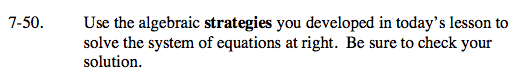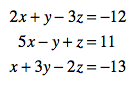Choose a pair of equations that can be added together to eliminate a variable.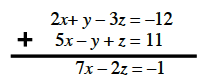Choose a different pair of equations to add together to eliminate the same variable.
In this case, you will have to multiply each equation by a constant.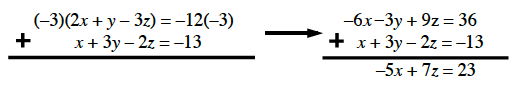Solve the system of equations you created in steps 1 and 2 for either x or z.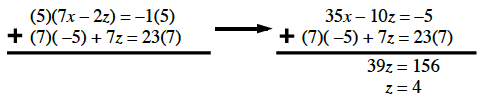Solve for x using the 2 variable equation you created in either step 1 or step 2.
Then substitute the values for x and z into one of the original equations to solve for y.

Check your solution in a different equation than the one you used to solve for y.